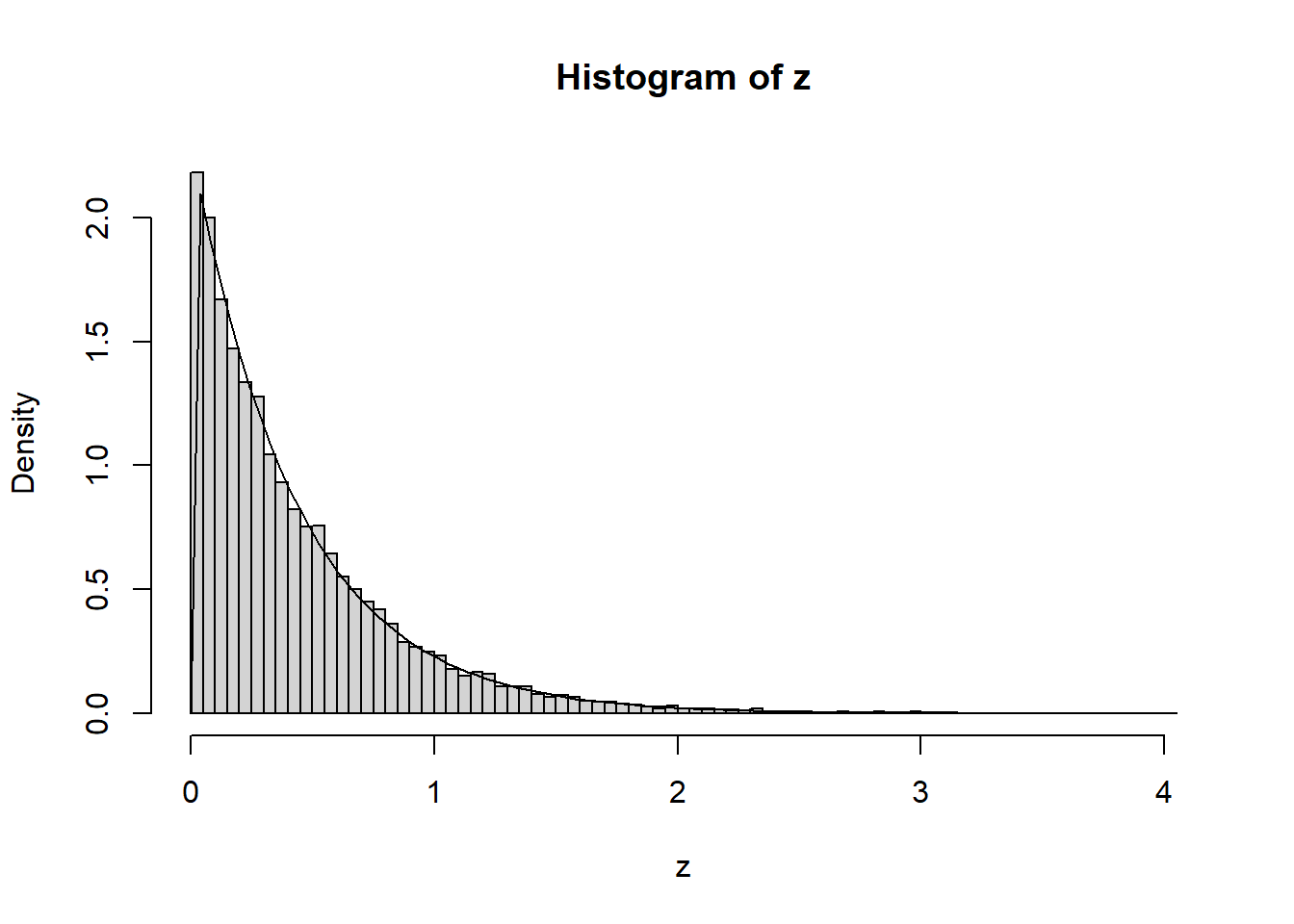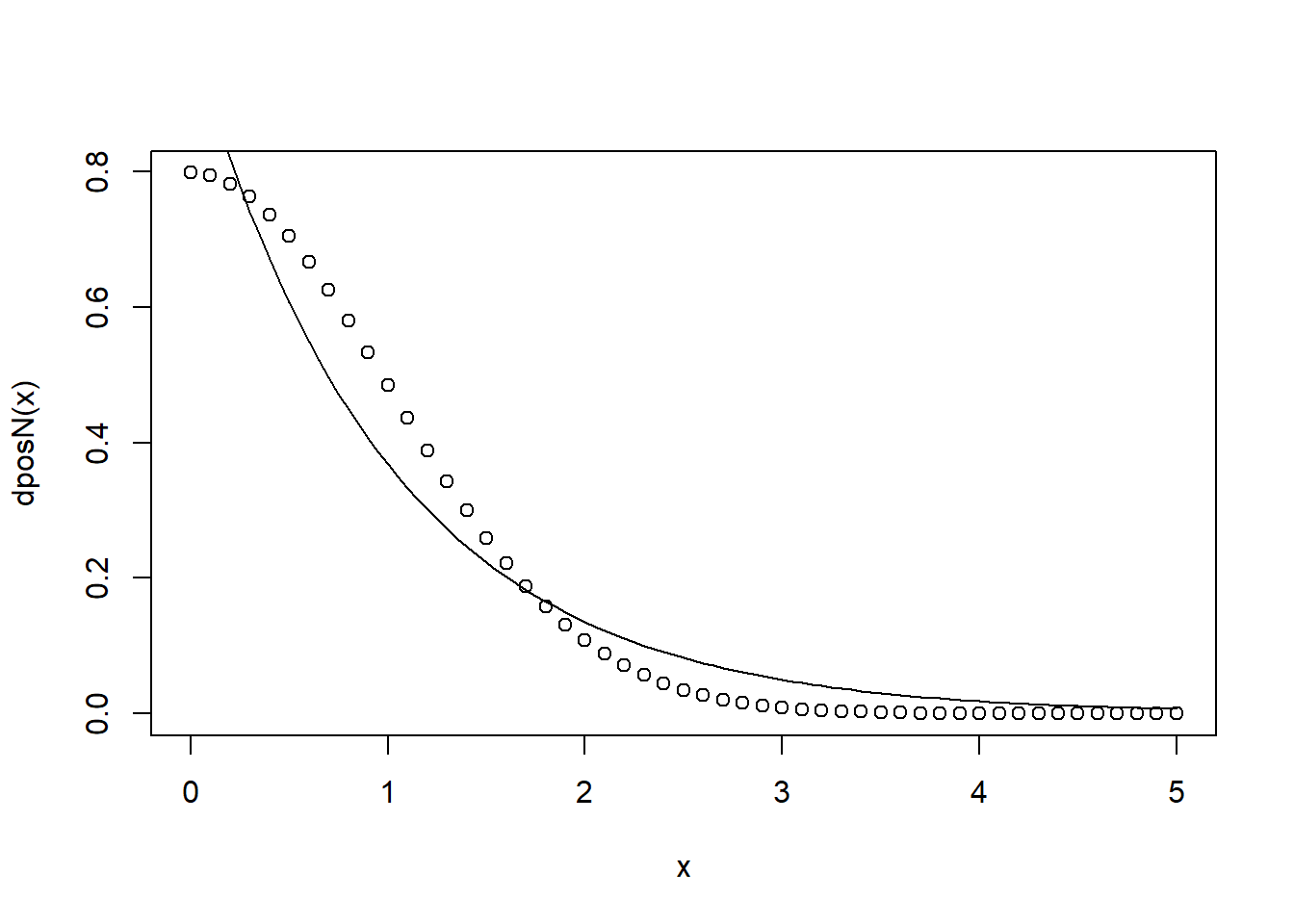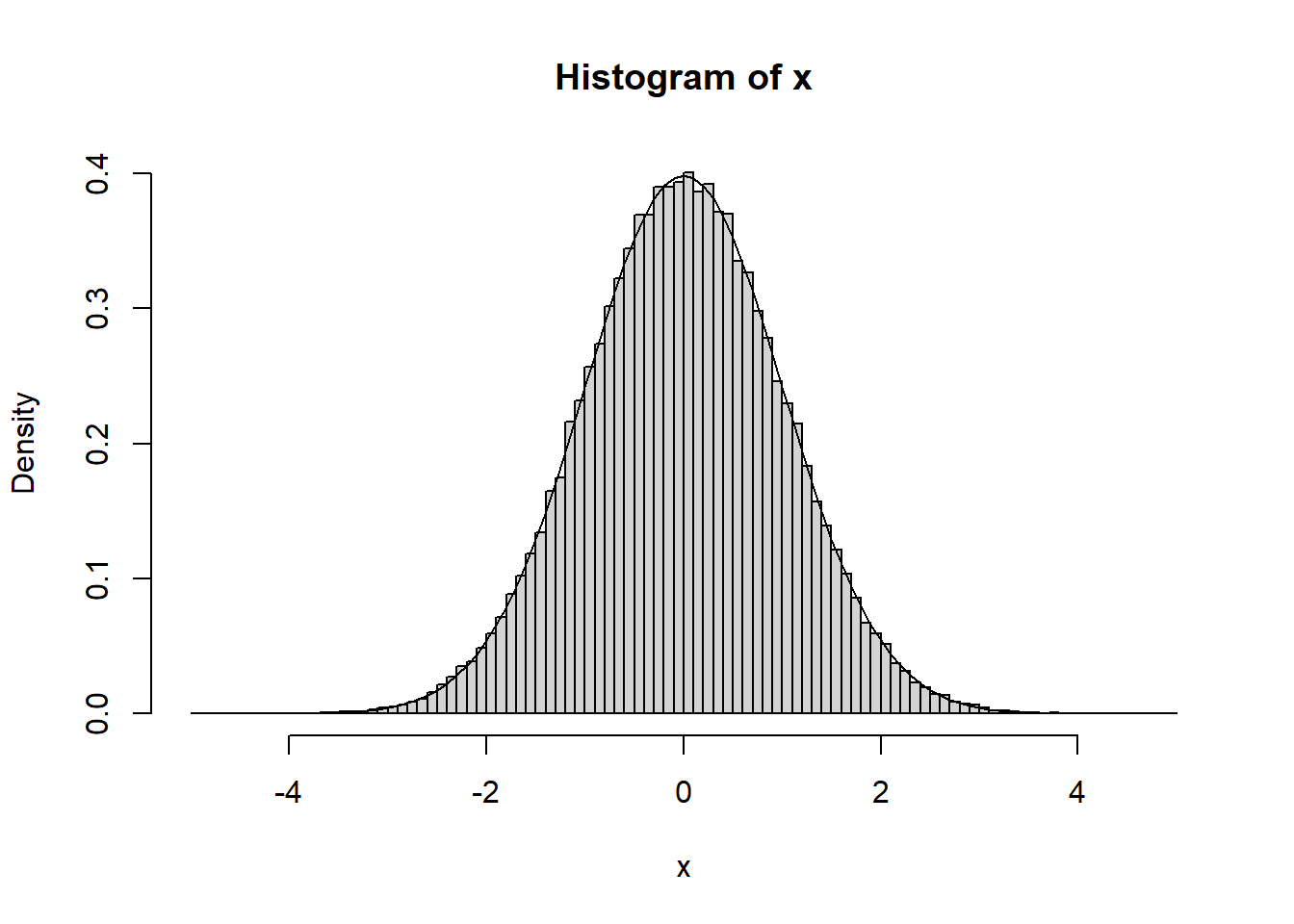# 1 Coding a Poisson random variable

Suppose R can only generate uniform random variables. How can you take advantage of this and generate Poisson random variables?

## 1.1 Solution

Let $$X$$ be a Poisson random variable of mean $$\lambda$$. Let $$p_i = \mathbb{P}(X =i)$$. We split the unit interval up into intervals $$J_i$$ of length $$p_i$$; this adds up to one, since the $$p_i$$ are a pmf. If $$U$$ is uniformly distributed on $$[0,1]$$, and $$U \in J_i$$, then we report $$X=i$$. This is accomplished in the R code below, and we perform various checks to make sure we didn’t mess up.

probpois <- function(x){
n = x
lambda = x
p = exp(-lambda) * (lambda^n) / factorial(n)
p
}

cumpois<- function(x){
n = x
lambda = x
sum=0
for(i in 0:n){
sum <- probpois( c(i, lambda)) + sum
}
sum
}

poisrv<- function(lambda){
u = runif(1)
m = -1
if( u < cumpois(c(0, lambda))){m <- 0}
i=1
while(m==-1){
if(u < cumpois(c(i,lambda))){m <-i}
i <- i+1
}
m
}

z= replicate(10000, poisrv(2.56))
mean(z)
##  2.547
var(z)
##  2.516443
sum(z==3)/10000
##  0.224
dpois(3, 2.56)
##  0.2161597

# 2 Inverse transform method

Use the inverse tranform method to generate an exponential random variable

## 2.1 Solution

Recall that if $$X$$ is exponential with rate $$\lambda$$, then $\mathbb{P}(X \geq x) = e^{-\lambda x}.$ Thus if $$F$$ is the cdf, its inverse has explicit formula given by $F^{-1} (y) = \frac{-\log (1-y)}{\lambda}.$

The inverse transform method tells that if $$U$$ is uniformly distributed on the unit interval then $$F^{-1}(U)$$ has the same as $$X$$. The R code below illustrates this fact.

lambda = 2.3
inverseT <- function(y){
inv =-log(1-y) / lambda
inv
}
x = runif(10000)
z = inverseT(x)
1/mean(z)
##  2.279966
hist(z, prob=TRUE, breaks=100)
curve(dexp(x,2.3), add=TRUE)# 3 The value of Pi

Inscribe a circle in a square. Estimate the value of $$\pi$$ by computing the ratio of the number of times a uniformly chosen point on the square ends up in the circle.

## 3.1 Solution

This ratio $$r$$ will tend towards the ratio of the area of circle to the area of square. We modify the code from the Random variables: theory and practice slides.

pointtrack <- function(N){
n=0
k=1
while(k<N+1){
x = 2*runif(1) -1
y = 2*runif(1) -1
if (x^2 + y^2 <1) { n<-n+1}
k<-k+1
}
n/N
}
pointtrack(100000)*4
##  3.14608

# 4 Acceptance/Rejection

Using an exponential random variable, generate a normal random variable that is conditioned to be be positive; from here, adjust this result to get a normal random variable.

## 4.1 Solution

Recall that the density of a standard normal is given $x \mapsto \frac{1}{\sqrt{2\pi}} e^{-x^2/2}.$

By symmetry, the density conditioned to be positive, is given by

$x \mapsto \frac{2}{\sqrt{2\pi}} e^{-x^2/2}.$

We can plot this against $$e^{-x}$$ the density of the exponential with rate $$1$$, to guess what $$M$$ to choose.

dposN <- function(x){
d = 2/ sqrt(2 * pi) * exp( -x^2/2)
d
}
x=seq(0, 5, by=0.1)
plot(x, dposN(x))
curve(dexp(x), add=TRUE)It is not hard to see we may take $$M=2$$.

ar <- function(){
x = -5
while(x==-5){
u = runif(1)
y = rexp(1)
w = dposN(y)/(2*dexp(y))
if( u < w){x <- y}
}
x
}
arfix<- function(){
x=(2*rbinom(1,1,0.5)-1)*ar()
x
}
x=replicate(100000, arfix())
z = seq(-5,5,0.1)
hist(x, prob=TRUE, z)
curve(dnorm, add=TRUE)# 5 Total variatonal distance

Let $$X$$ and $$Y$$ be Poisson random variables with means $$\lambda > \mu$$. Show that $d_{TV}(X, Y) \leq 2 (1-\exp( \mu-\lambda))$

## 5.1 Solution

Recall that if $$Z$$ is Poisson with mean $$\lambda - \mu$$ and independent of $$Y$$, then $$X'=Z + Y$$ is a Poisson random variable with mean $$\lambda$$. Thus $d_{TV}(X, Y) = d_{TV}(X', Y) \leq 2\mathbb{P}(X' \not =Y) = 2 \mathbb{P}(Z >0) = 2 (1-\exp( \mu-\lambda)).$

# 6 Reversibility

Let $$P$$ be transition matrix on a state space $$S$$, and $$\pi$$ be a probability measure on $$S$$. We say that $$\pi$$ is reversible for $$P$$ if $$\pi_i p_{ij} = \pi_j p_{ji}$$.

• Check that the stationary distribution from a random walk on a finite graph is reversible.

• Let $$P$$ be a transition matrix on a state space $$S$$. Check that if $$\pi$$ is reversible, then it is stationary.

• Let $$\pi$$ be a reversible distribution for the transition matrix $$P$$ on a state space $$S$$. Let $$X$$ be Markov chain with transition matrix $$P$$, that is started at $$\pi$$. Let $$a, b,c,d \in S$$. Show that $\mathbb{P}(X_0=a,X_1=b, X_2=c, X_3=d) = \mathbb{P}(X_0=d,X_1=c,X_2=b, X_3=a).$

## 6.1 Solution

• Let $$G=(V, E)$$ be a simple undirected graph. Recall that $$\pi_i = \deg(i)/ 2|E|$$, and the transition matrix $$P$$ is given by $p_{ij}=\frac{1}{\deg(i)}\mathbf{1}[(i,j) \in E].$ We have the following calculation: $\begin{eqnarray*} \pi_i p_{ij} &=& \frac{1}{2|E|}\mathbf{1}[(i,j) \in E] \\ &=& \frac{\deg(j)}{2|E|} \Big(\frac{1}{\deg(j)}\Big)\mathbf{1}[(j,i) \in E] \\ &=& \pi_j p_{ji}. \end{eqnarray*}$

• We have $\begin{eqnarray*} (\pi P)_j &=& \sum_{i \in S} \pi_i p_{ij} \\ &=& \sum_{i \in S} \pi_j p_{ji} = \pi_j \sum_{i \in S} p_{ji} \\ &=& \pi_j. \end{eqnarray*}$

• We have by reversibility that

$\begin{eqnarray*} \mathbb{P}(X_0=a,X_1=b, X_2=c, X_3=d) &=& [\pi(a)p_{ab}] p_{bc} p_{cd} \\ &=& p_{ba} [ \pi(b)p_{bc} ] p_{cd} \\ &=& p_{ba} p_{cb} [\pi(c) p_{cd} ] \\ &=& p_{ba} p_{cb} p_{dc} \pi(d) \\ &=& \pi(d) p_{dc} p_{cb} p_{ba} \\ &=& \mathbb{P}(X_0=d,X_1=c,X_2=b, X_3=a), \end{eqnarray*}$ as desired.

# 7 Simple card shuffling

Suppose that I have $$n=52$$ cards, arranged in some initial order. Consider the following procedure: I choose two cards with probability $$1/ {n \choose 2}$$, and then change their position; repeat.

• Describe this procedure as a Markov chain. Can you code it?

• With this procedure, can you get from an initial ordering to any other ordering? Why?

• Do you think it has a stationary distribution? If, so, what is it?

## 7.1 Solution

• Recall that $$S_n$$ is the group of all permutations of $$[n]=\{1, 2, \ldots, n\}$$; that is, $$g \in S_n$$ if and only if $$g$$ is bijection from $$[n]$$ to itself. Group multiplication, is given by function composition, so that we write $$gh = g\circ h$$. Recall that a transposition $$g \in S_n$$, is a permutation such that there exists two elements $$i,j \in [n]$$ such that $$g(i) = j$$, and for all other elements $$x \in [n]$$ are fixed; that is, $$g(x) =x$$. Let $$X$$ be a Markov chain on $$S_n$$ with transition probabilities given by $\mathbb{P}(X_1= \sigma g\ | \ X_0 = g) = 1 / {n \choose 2}$ for every transposition $$\sigma \in S_n$$.

• Yes. Recall that every permutation is a product of transpositions. For example, consider the case $$n=5$$, and $$g(1)=2, g(2)=5$$, $$g(3)=1$$, $$g(5)=3$$, and $$g(4)=4$$. This is usually graphically displayed via $$g=(1253).$$ Note that $g= (12)(25)(53).$

• The uniform distribution $$\pi$$ on $$S_n$$ assigns probability $$1/n!$$ to each of the $$n!$$ elements of $$S_n$$ is clearly stationary.

deck = seq(1,52, 1)
deck
##    1  2  3  4  5  6  7  8  9 10 11 12 13 14 15 16 17 18 19 20 21 22 23 24 25
##  26 27 28 29 30 31 32 33 34 35 36 37 38 39 40 41 42 43 44 45 46 47 48 49 50
##  51 52
shuffle <- function(x){
t=sample(52, 2, replace=FALSE)
a=t
b=t
da = x[a]
db = x[b]
x[a] <- db
x[b] <- da
x
}
y=shuffle(deck)
y
##    1  2  3  4  5  6  7  8  9 10 11 12 13 14 28 16 17 18 19 20 21 22 23 24 25
##  26 27 15 29 30 31 32 33 34 35 36 37 38 39 40 41 42 43 44 45 46 47 48 49 50
##  51 52
shuffle(y)
##    1  2  3  4  5  6  7  8  9 10 11 12 13 14 28 16 17 18 19 20 21 22 43 24 25
##  26 27 15 29 30 31 32 33 34 35 36 37 38 39 40 41 42 23 44 45 46 47 48 49 50
##  51 52
for( i in 1:100){
y <- shuffle(y)
}
y
##   31 27  8  4 25  5 39 35 17 52 29 26  1 41 13 33 14 45 11 30  6 10 44 43 46
##  24 50 42 36 34 38 32 47  2 23 51 16 15 48  3 18 49 12 20 37 21 40 19 28 22
##   9  7